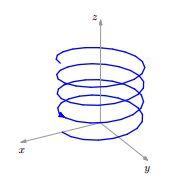# Length Of A Helix

Calculus Level 4Consider the cylindrical helix in $\mathbb{R}^3$ whose equations are

$x = 3\cos(t) \hspace{.4cm} y = 3\sin(t) \hspace{.4cm} z = 4t$

What is the length of this helix from $t = 0$ to $t = 403$?

×